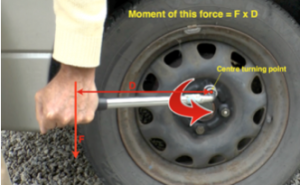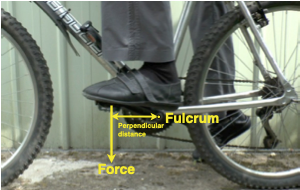### What is a moment

The moment of a force is used to measure how hard something is being turned or twisted about a turning point. It depends upon how big the force is and on how long the lever is. The turning point is often called the pivot or fulcrum.Click below to download a PDF document of notes on The Law of Moments and some applications including calculations:

Moments and the law of moments notes PDF

Download PDF of all notes on The Law of Moments  Moments and the law of moments notes

### Calculation of a moment

The moment of a force is the perpendicular distance to the line of the force multiplied by the size of the force.

Measured in newton metres (Nm).

Moment of a force (Nm) = force(N) x perpendicular distance(m)### Measuring the perpendicular distance

The perpendicular distance is the shortest distance from the pivot to the line of direction of the force.